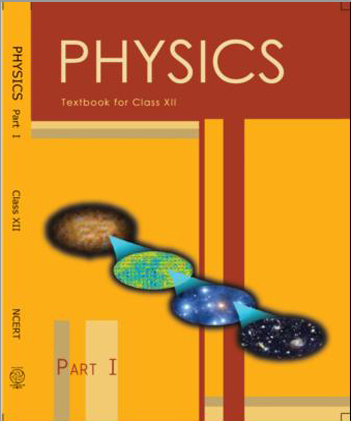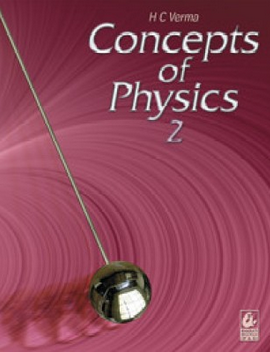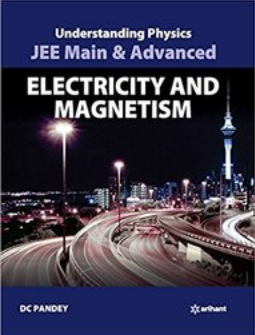# Current Electricity   Share

## What is Current Electricity

Look around you and you will realize that you are surrounded with many electronic gadgets like mobile phone, TV or laptop etc. All of these devices will stop working if they won’t have an energy source or a battery. This energy comes in the form of electric current. Not only that nature also has many types of currents like the lightning in the clouds is a result of the uncontrolled flow of charges to earth.## Topics of Current Electricity

Electric current, Drift velocity, Ohm’s law, Electrical resistance, Resistances of different materials, V-I characteristics of Ohmic and non-ohmic conductors, Electrical energy and power, Electrical resistivity, Colour code for resistors; Series and parallel combinations of resistors; Temperature dependence of resistance. Electric Cell and its Internal resistance, potential difference and emf of a cell, Combination of cells in series and in parallel. Kirchhoff’s laws and their applications. Wheatstone bridge, Metre bridge. Potentiometer – principle and its applications.

## Overview of Current & Electricity

This chapter talks mainly about electric current and potential difference. So what basically is this electric current? Current is the measure of the flow of charge, and it is represented by I where,. In this chapter, we will concentrate mainly about electric current in metals, because in metals, the electrons are free to move and when an Electric field is applied or potential difference is created in the conductor, the electrons flow and hence current flows in the metals, these metals are known as conductors. Few examples of good conductors are gold, silver, copper, and aluminum. In the conductors, the potential difference (V) is directly proportional to the current (I). This relation is known as Ohm’s law and it was given by G.S. Ohm in the year 1828. The proportionality constant of this relation is also known as resistance and it is represented by R. This constant R depends upon the material characteristics as well as its dimensions. Due to dependency on the dimension, two or more resistance can form 3 types of arrangements namely series, parallel and mixture of both. In this chapter, you will mainly find questions for calculating the equivalent resistance of various arrangements of resistances and two laws of Kirchhoff’s will help you to find it, namely, Kirchhoff’s Junction rule and Kirchhoff’s Loop rule. There are some interesting experiments which you will have or had done in your school like Potentiometer experiment, Wheatstone bridge or meter bridge experiments. In this chapter, you will also get to know about the working of various electrical measuring devices, mainly about the galvanometer, ammeter, and voltmeter.

Prepare Online for JEE Main/NEET

Crack JEE 2021 with JEE/NEET Online Preparation Program

## Formulas for Current & Electricity

• Drift Velocity,whereis relaxation time & E is Electric Field

• Current in terms of drift velocity,where n is free e- density.

• Current density,• Ohm’s law,• Resistance,whereis resistivity

• Equivalent Resistance

• Parallel arrangement,• Series arrangement,• Power,• Kirchhoff’s junction rule,• Kirchhoff’s loop rule,## How to prepare Current Electricity

Before solving questions of this chapter you should be well versed with the concepts of electrostatics, you should be able to find the equivalent capacitance and calculate the potential difference. This chapter is easy if you know the concepts of electrostatics. You will find many questions of this chapter which involve your practical knowledge, like questions on potentiometer, ammeter or voltmeter etc, so do your practicals very carefully and understand each and everything about it. If you are not able to do practicals then watch animated videos of working of all these electronic measuring devices. Many times you will be given complex circuits of resistors and you either have to solve for equivalent resistance, current or potential difference. So keep practicing questions on circuits.

## Notes for Current & Electricity

• Before solving questions of this chapter, revise concepts of electrostatics, especially concepts related to capacitance.

• Try to solve questions with the help of Kirchhoff’s rule, it will be helpful for you while solving complex circuit questions.

• Do your lab experiments carefully, most often questions are asked from these experiments as well.

• Solve as many questions of complex circuit questions as you can, and if your textbook doesn’t have enough questions, then give mock tests.

• Make a list of questions which you were not able to solve in the first attempt or you took a long time solving them, after a week or two solve them again.

## Books for Current Electricity

For this chapter, I would recommend you to first go through NCERT book and your Lab manual and solve questions from those chapters. Then you should solve questions from NCERT Exemplar book for a good hold on this chapter. If you want to test yourself for competitive exams, then you should read Understanding Physics by D.C. Pandey.

## Physics Chapter wise Notes For Engineering and Medical Exams

 Chapters No. Chapters Name Chapter 1 Physics and Measurement Chapter 2 Kinematics Chapter 3 Laws of motion Chapter 4 Work Energy and Power Chapter 5 Rotational Motion Chapter 6 Gravitation Chapter 7 Properties of Solids and Liquids Chapter 8 Kinetic theory of Gases Chapter 9 Thermodynamics Chapter 10 Oscillations and Waves Chapter 11 Electrostatics Chapter 12 Current Electricity Chapter 13 Magnetic Effects of Current and Magnetism Chapter 14 Electromagnetic Induction and Alternating currents Chapter 15 Electromagnetic Waves Chapter 16 Optics Chapter 17 Dual Nature of Matter and Radiation Chapter  18 Atoms And Nuclei Chapter 19 Electronic devices Chapter 20 Communication Systems Chapter 21 Experimental skills

### Topics from Current Electricity

• Electric currents ( JEE Main ) (21 concepts)
• Drift velocity ( JEE Main ) (9 concepts)
• Ohm's laws ( JEE Main ) (5 concepts)
• Electrical resistance, Resistances of different materials ( JEE Main ) (6 concepts)
• Electrical resistivity, Colour code for resistors; ( JEE Main ) (22 concepts)
• Series and parallel combinations of resistors ( JEE Main ) (9 concepts)
• Electric Cell and its Internal resistance ( JEE Main ) (23 concepts)
• the combination of cells in series and in parallel. ( JEE Main ) (37 concepts)
• Kirchhoff's laws and their applications ( JEE Main ) (13 concepts)
• Wheatstone bridge ( JEE Main ) (3 concepts)
• Metre bridge ( JEE Main ) (3 concepts)
• Potentiometer: principle and its applications. ( JEE Main ) (6 concepts)
• Temperature dependence of resistance. ( JEE Main ) (2 concepts)
• Electric Current ( JEE Main ) (3 concepts)
• Ohms Law ( JEE Main ) (3 concepts)
• Resistance and Resistivity ( JEE Main ) (3 concepts)
• Heat and Power ( JEE Main ) (1 concepts)
• Series and Parallel Grouping of Resistance ( JEE Main ) (2 concepts)
• Cell and Combination of Cell ( JEE Main ) (6 concepts)
• Kirchhoff Law ( JEE Main ) (5 concepts)
• Wheatstone's Bridge and Meter Bridge ( JEE Main ) (2 concepts)
• Potentiometer ( JEE Main ) (5 concepts)
• Faraday's Law of Electrolysis ( JEE Main ) (1 concepts)
• Thermo Couple ( JEE Main ) (1 concepts)
• Resistance of Complex Circuit ( JEE Main ) (1 concepts)
• Charging of Capacitors and Inductors ( JEE Main ) (2 concepts)

### Important Books for Current Electricity

•••Exams
Articles
Questions